# Audio Cats and Dogs

Dataset consisting of recordings of cats and dogs

The dataset contains 164 recordings of cat sounds (1,323 seconds) and 113 recordings of dog sounds (598 seconds). The length of the recordings is variable.

## Examples

### Basic Examples

Retrieve the resource:

 In:=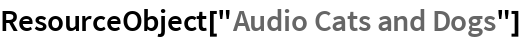Out=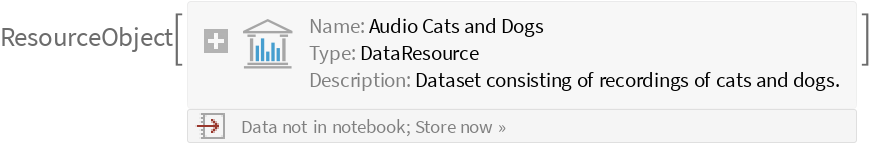Retrieve the dataset:

 In:=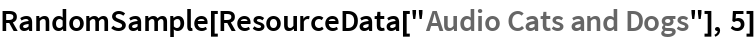Out=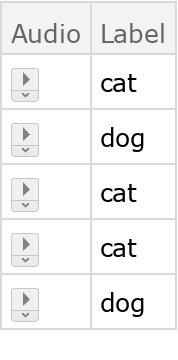### Visualization

Select an entry from the dataset:

 In:=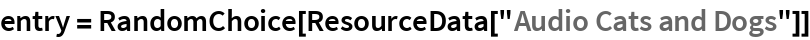Out=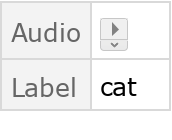Visualize the waveform of the Audio object:

 In:=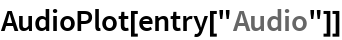Out=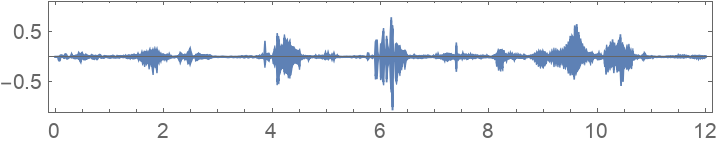Visualize the spectrum:

 In:=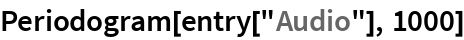Out=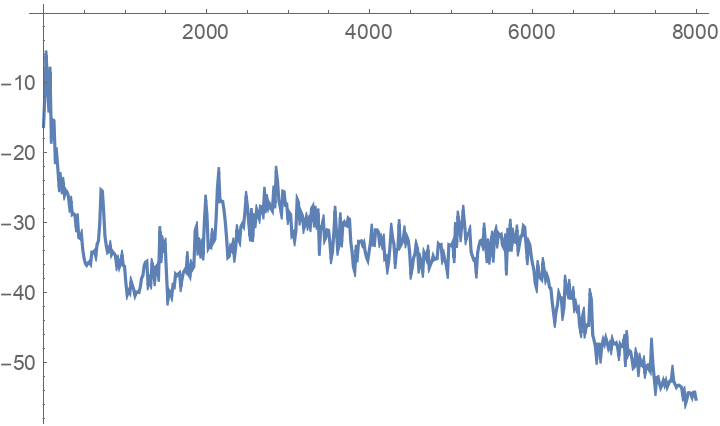Visualize the spectrogram:

 In:=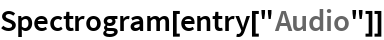Out=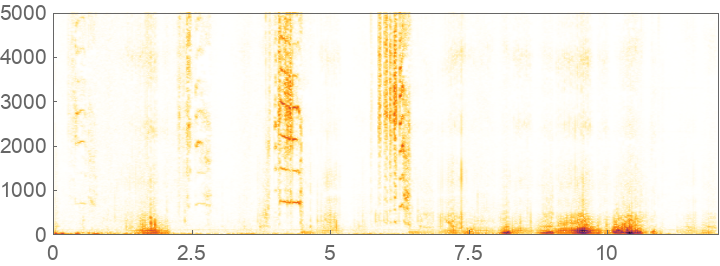### Analysis

Compute the number of examples per class:

 In:=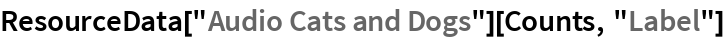Out=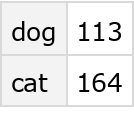Inspect the sample rate and channel count of the Audio objects:

 In:=Out=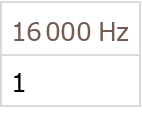Plot the histogram of the durations of the Audio objects:

 In:=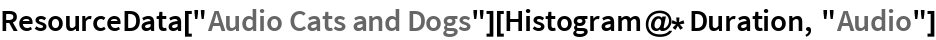Out=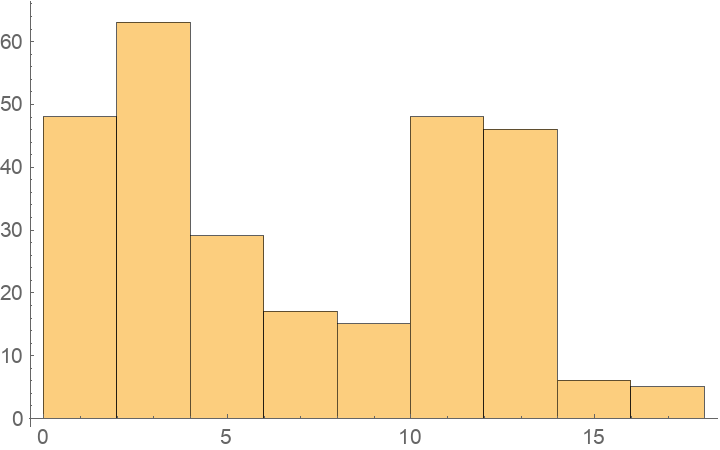By computing the cumulative duration of the labels, it can be seen that the dataset is not balanced:

 In:=Out=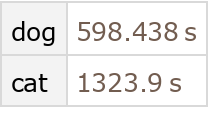The average recording duration for the two labels is different as well:

 In:=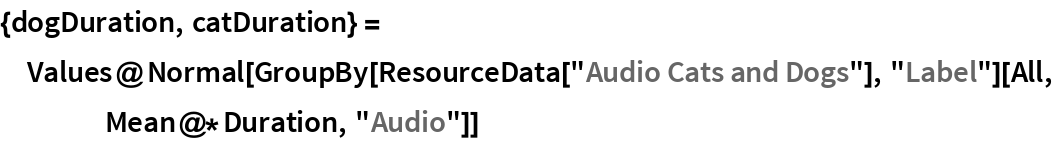Out=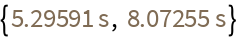Create a balanced training dataset.

 In:=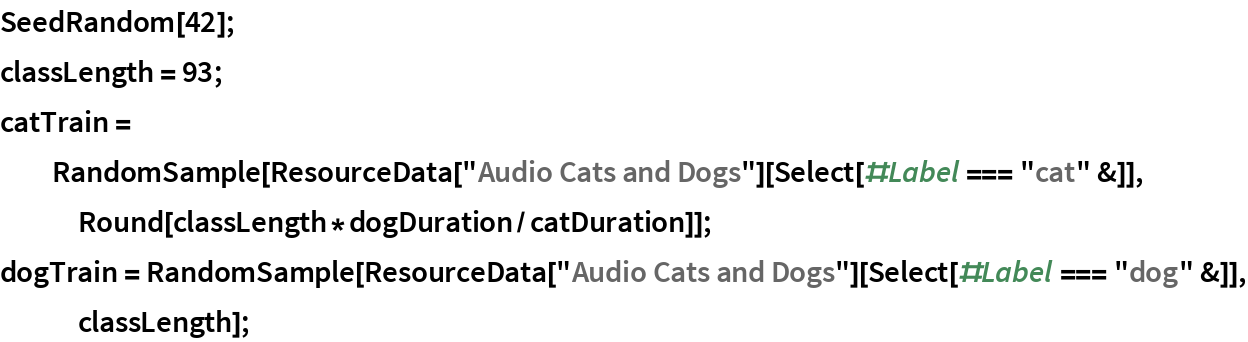Make sure that the class total duration of the selected samples is similar:

 In:=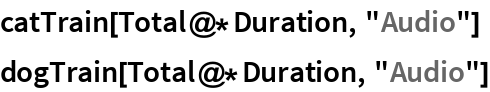Out=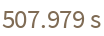Out=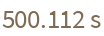Join the two classes and shuffle the elements:

 In:=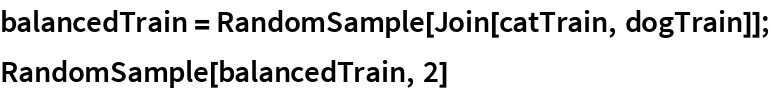Out=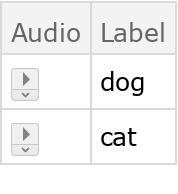Create an unbalanced testing dataset using the remaining data:

 In:=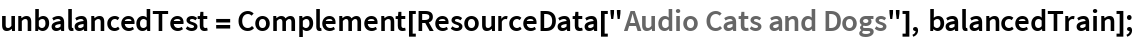In:=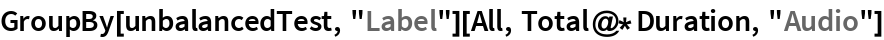Out=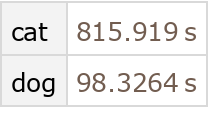Wolfram Research, "Audio Cats and Dogs" from the Wolfram Data Repository (2018)